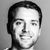# App Development

Discussion board where members can learn more about Qlik Sense App Development and Usage.

Announcements
Welcome to Qlik Community! Check out our new navigation! FIND OUT MORE
cancel
Showing results for
Did you mean:Contributor III

## Aggr for 2x dimensions - sorting by expresion problem (Pareto analysis)

Hi, I want to calculate running total % of sales for pareto analysis. Everything works fine when working with one dimension. Lets say i have dimension "Key" and wanna to sort it by "sum(Sales)" in descending order using Aggr. So i do:

```Aggr(
rangesum(Above(sum([Sales])/sum(TOTAL [Sales]), 0, rowno() )),
(Key, (=Sum([Sales]), DESC))
)```

That just works. But when i want to put into a play second dimension (lets say month) things is starting to get messy. First i went with this set analysis:

```=Aggr(
rangesum(Above(sum([Sales])/sum(TOTAL <Month> [Sales]), 0, rowno() )),
Month, (Key, (=Sum([Sales]), DESC))
)```

The problem with the above is that Aggr sorts rows within Aggr by Key, but GLOBALLY not by each month SEPARETLY. Which leads to incorrect results. Is there any way to sort data within Aggr by Key & Month?

I came up with an ugly solution, thats is not flexible in any means (requires loading script changes). I just loaded every possible combination of Key & Month into my model and created new dimension KeyMonthCombined. Then expression looks like:

```=Aggr(
rangesum(Above(sum([Sales])/sum(TOTAL <Month> [Sales]), 0, rowno() )),
Month, (KeyMonthCombined, (=Sum([Sales]), DESC))
)```

And it works. But i feel like I'm putting too much effort into this and it could be done in much more easy way.

Thanks!

3 RepliesContributor III
Author

Anyone?Contributor III
Author
I would really like an opinion about the above. App performance is at unacceptable level when using my workaround. Really noone studied sorting by expression inside Aggr() ??Creator III

Hi @BeeGees, I'm facing the exact same problem. Have you been able to find a solution?Tags
Community Browser